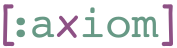## 1    unify-fn: Unifying Input

Unification plays a key part in logic programming. The term comes from mathematical logic, where it refers to the most basic form of equation known in mathematics. This is a purely syntactic or structural equality. For example the equation `x+3=5` does not have a solution because unification does not know the meaning of `+`. However, the equation `x+3=2+y` has a single solution: `x=2` and `y=3`. In logic programming, unification achieves two things:

1. It checks if the input conforms to some expected pattern, and
2. If so, it binds values from the input to variables in the pattern.

While mathematical unification is symmetric, and and so are most other implementations (e.g., Prolog and core.logic), our implementation of unification is asymmetric. We treat the pattern and the input as two different things.

The `unify-fn` macro takes the following arguments:

1. A collection of free arguments.
2. A pattern consisting of nested sequential elements (`seq`, `vec`), containing symbols (out of the free variable list)and possibly other values.
3. An expression to be evaluated with the bindings.It evaluates to a function that takes an input value and returns a sequence.
4. If the input matches the pattern, the returned sequence will contain a single element – the evaluation of the expression under the bindings.
5. If the input does not match the pattern, the sequence will be empty.
``````((unify-fn [x y] [x y] (+ x y)) [1 2]) => 
(let [f (unify-fn [x] [x 2] (+ x 2))]
(f [1 2]) => 
(f [1 3]) => empty?)
(let [f (unify-fn [x y] [:foo [:bar x] [:baz y +]] [x y])]
(f [:foo [:bar 3] [:baz 5 +]]) => [[3 5]]
(f [:foo [:bar 3] [:baz 5 -]]) => empty?)``````

### 1.1    Under the Hood

The function `at-path` returns a Clojure expression that evaluates a path in a tree. For example:

``(at-path 'tree [1 2 3]) => '(((tree 1) 2) 3)``

`traverse` takes a tree and a predicate function as input and returns a map from paths to nodes.

``````(traverse 1 (constantly true)) => {[] 1}
(traverse [1 [2 3]] (constantly true)) => { 1
[1 0] 2
[1 1] 3}``````

The predicate function tells `traverse` whether to enter a sub-tree or not. In the following example, we enter only vectors and treat all non-vector elements as monoliths.

``````(traverse '[1 (2 3) [4 (5 6)]] vector?) => { 1
 '(2 3)
[2 0] 4
[2 1] '(5 6)}``````

From a map produced by `traverse` we create two artifacts:

1. Bindings, according to variables that appear in the map, and
2. Conditions, according to values that appear in the map.

The function `conds-and-bindings` takes the entries of the map produced by `traverse` and a predicate that determines if a sub-tree is a value or a variable and returns a list of conditions and a list of bindings.

``````(conds-and-bindings [] (constantly true)) => [[] []]
(conds-and-bindings [[ 'x] [ 'y]] (constantly true))
=> `[[] [~'x (~'\$input\$ 0)
~'y (~'\$input\$ 1)]]
(conds-and-bindings [[ 'x] [ "foo"]] symbol?)
=> `[[(= (~'\$input\$ 1) "foo")]
[~'x (~'\$input\$ 0)]]``````